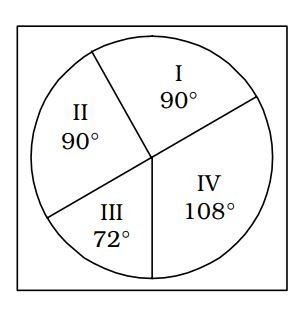## Introduction to Data Interpretation

#### Data Interpretation

Direction: Study the pie chart and answer the given questions. The total expenditure of a company for a particular month is Rs. 60000. The various heads of expenditure I to IV are indicated in a pie chart given below. These heads are :
I. Raw materials
II. Conveyance
III. Electricity1. What percentage of total expenditure is on electricity?

1. According to given pie chart in question , Corresponding angle for expenditure on electricity = 72°
Total angle for expenditure = 360°
Total percent for expenditure = 100%
⇒ 360° ≡ 100%
We know that ,

 ∴ Required percent = Total percent for expenditure × Corresponding angle for expenditure on electricity Total angle for expenditure

##### Correct Option: D

According to given pie chart in question , Corresponding angle for expenditure on electricity = 72°
Total angle for expenditure = 360°
Total percent for expenditure = 100%
⇒ 360° ≡ 100%
We know that ,

 ∴ Required percent = Total percent for expenditure × Corresponding angle for expenditure on electricity Total angle for expenditure

 ∴ Required percent = 72° ≡ 100 × 72 = 20% 360

Direction: Study the Pie chart carefully and answer the questions.1. The number of students who are members of both the clubs is :

1. As per the given pie chart in question ,
Percent of non - members = 5%
Number of members of non - members = 40
∴ 5% ≡ 40
Percent of members of both the clubs = 75%

##### Correct Option: D

As per the given pie chart in question ,
Percent of non - members = 5%
Number of members of non - members = 40
∴ 5% ≡ 40
Percent of members of both the clubs = 75%

 ∴ 75% ≡ 40 × 75 = 600 5

Hence , Number of members of both the clubs is 600

1. Ratio of members of cricket club only and football club only respectively is :

1. From given above pie chart ,
Percent of cricket club only = 5%
Percent of football club only = 15%
Required ratio = Percent of cricket club only : Percent of football club only

##### Correct Option: A

From given above pie chart ,
Percent of cricket club only = 5%
Percent of football club only = 15%
Required ratio = Percent of cricket club only : Percent of football club only
∴ Required ratio = 5 : 15 = 1 : 3

1. Number of students who are members of cricket club only :

1. From given above pie chart ,
Percent of Non–members = [ 100 – ( 75 + 15 + 5 ) ]% = 5%
Number of Non–members = 40
⇒ 5% = 40 ........( 1 )
Percent of cricket club only = 5%
⇒ Members of cricket club only = 40 { From ( 1 ) }

##### Correct Option: B

From given above pie chart ,
Percent of Non–members = [ 100 – ( 75 + 15 + 5 ) ]% = 5%
Number of Non–members = 40
⇒ 5% = 40 ........( 1 )
Percent of cricket club only = 5%
⇒ Members of cricket club only = 40 { From ( 1 ) }
Hence , Number of students who members of cricket club only are 40.

1. Percentage of students who are not members of any club is :

1. On the basis of given pie chart in question ,
Total percent of students = 100%
Members of only football club = 15%
Members of only cricket club = 5%

##### Correct Option: A

On the basis of given pie chart in question ,
Total percent of students = 100%
Members of only football club = 15%
Members of only cricket club = 5%
Members of both football and cricket club = 15%
Required percent = [ 100 – ( 75 + 15 + 5 ) ]% = 5%